Print version

Before a microwave link can be installed, an analysis and calculation of the microwave link must be made first. The analysis should take place before the site survey itself to get a clear idea about the dimensions of the antennas. The analysis consists of the following steps:

• Free space loss calculation

• Rain attenuation

• Fresnel zones calculation

This chapter explains the individual steps and an example of link design is given at the end.

NOTE: For quick reference you can use the calculator on www.racom.eu

## 1.1. Free space loss calculation

As the electromagnetic waves travel through open space they are attenuated. This attenuation is described as Free-space Loss. The loss depends on the distance travelled by signal and its frequency. Longer distance and higher frequency both mean greater attenuation. Free-space loss can be calculated thus:

FSL = 32.44 + 20log f + 20log D

Where:

 FSL free-space loss (dB) f frequency of the emitted signal (MHz) D length of the link (km)

If we ignore the additional loss along the path, the received signal strength can be calculated using the formula for signal propagation in free space as follows:

PR = PT + GT + GRFSL

Where

 PR received power level (dBm) PT transmitted power (dBm) GT transmitting antenna gain (dBi) GR receiving antenna gain (dBi) FSL free space loss (dB)

PR must be:

PR > PS

Where:

The receiver’s sensitivity defines the minimum level of the received signal at which the receiver is able to process the signal without losses or affecting the transmitted data (for BER better then 10-6).

Determining sufficient fade margin is the most important step in microwave link design. If the margin is too small, the link will be unstable – as a result, sufficient availability of the link or quality of the provided services cannot be guaranteed. On the other hand, unnecessarily large margin makes the link more expensive (higher performance, larger and more expensive antennas) and increases the cost of creating the microwave link.

The following paragraphs describe the two most significant types of signal strength loss – rain and multipath attenuation, which are the most frequent along with free space loss. Mutual relation between rain and multipath attenuation rules out the possibility that the link could be affected by both types of attenuation at the same time – these types of attenuation do not add up. To determine the fade margin it is necessary to calculate both rain and multipath attenuation. The larger of the two types of attenuation determines the value of fade margin. In areas with high precipitation, rain attenuation can be expected to be more prominent. By contrast, links located in drier climates and little inclination, will suffer more from multipath attenuation.

## 1.4. Rain attenuation

For frequencies of about 10 GHz rain attenuation starts to become increasingly effective. Precipitation is not identical in all areas which is why ITU released a recommendation Rec. ITU-R PN.837-1 for splitting the world into 15 regions according to precipitation intensity see Fig. 1.1, for more detail Appendix A, Rain zone map. In the areas with higher precipitation greater rain attenuation must be expected and a greater signal fade margin must be established; see the calculation of link availability.

The following properties are inherent to rain attenuation:

• It increases exponentially with rain intensity

• It becomes significantly larger as the distance travelled increases (>10 Km)

• Horizontal polarization causes greater rain attenuation than vertical polarization

• Rain outage increases dramatically with frequency and path lengthFig. 1.1: Rain zone map, based on Rec.ITU-R PN.837-1

Rain attenuation can be calculated using ITU-R outage model, which consists of the following:

Obtain the rain rate R0.01 exceeded for 0.01 per cent of the time (with an integration time of 1 min). R0.01 values are defined for 15 rain zones and different time percentages and they are given in ITU-R Recommendation P.837.

Tab. 1.1: Rain rate R (mm/h) ITU-R P.837

Percentage of time (%)ABCDEFGHJKLMNPQ
1.0<0.10.50.72.10.61.7328152451214
0.30.822.84.52.44.5741342711153449
0.1235868121020121522356572
0.035691312152018282333406510596
0.018121519222830323542606395145115
0.0031421262941544555457010595140200142
0.001223242427078658355100150120180250170

Compute specific attenuation γR (dB/km) for the frequency, polarization, specific rain rate using ITU-R recommendation P.838. Rain attenuation for rain rate γR0.01 can be calculated as follows:

γR0.01 = kh,v .R0.01αh,v

where:

 kh,v, αh,v constants for horizontal and vertical polarization. Constants are slightly different for each polarization, see next table according to ITU-R P.838

Tab. 1.2: Constants k, α for horizontal and vertical polarization at 10, 11, 17 and 24 GHz

 kh αh kv αv 10 GHz 0.01 1.26 0.01 1.22 11 GHz 0.02 1.21 0.02 1.16 17 GHz 0.06 1.09 0.07 1.01 24 GHz 0.14 1.01 0.14 0.96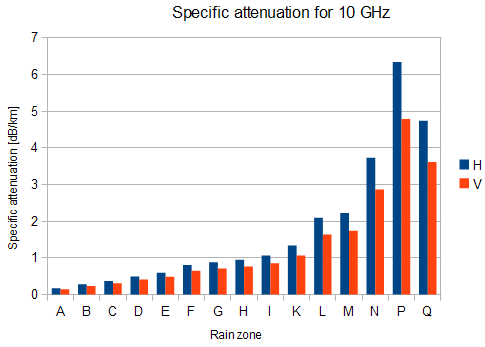Fig. 1.2: Attenuation for 10 GHz,
polarization H, V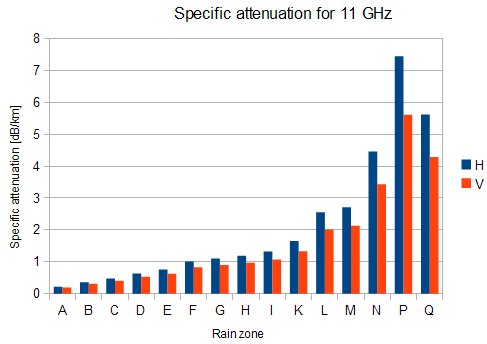Fig. 1.3: Attenuation for 11 GHz,
polarization H, V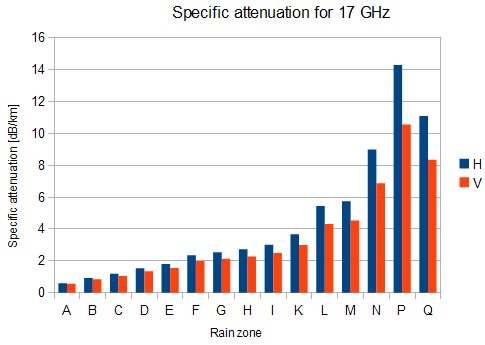Fig. 1.4: Attenuation for 17 GHz,
polarization H, V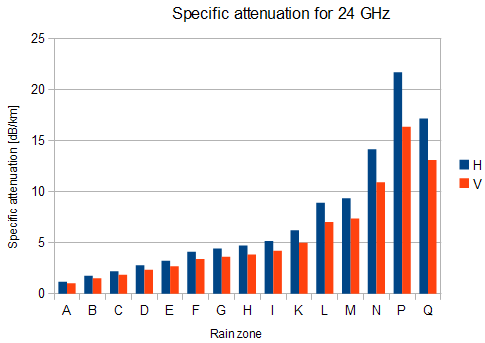Fig. 1.5: Attenuation for 24 GHz,
polarization H, V

Fig. 1.2 shows that rain attenuation is greater for horizontal polarization. In regions with higher precipitation the difference in attenuation is more marked. The microwave links RAy17 and RAy24 use both polarizations, hence the need to consider the worse of the two, i.e. horizontal polarization. When ACM is active we recommend using horizontal polarization in the direction with lower data traffic (typically up-link).

Multipath fading is another dominant fading mechanism. A reflected wave causes a phenomenon known as multipath, meaning that the radio signal can travel multiple paths to reach the receiver. Typically, multipath occurs when a reflected wave reaches the receiver at the same time in opposite phase as the direct wave that travels in a straight line from the transmitter.

Multipath propagation gives rise to two kinds of signal degrading effects, i.e., flat fading and frequency selective fading. Flat fading is a reduction in input signal level where all frequencies in the channel of interest are equally affected and is dependent on path length, frequency, and path inclination. In addition, it is strongly dependent on the geoclimatic factor K.

To calculate the probability of outage due to multipath propagation of microwave links the ITU-R probability model can be used which describes a single frequency (or narrowband) fading distribution suitable for large fade depths A in the average worst month in any part of the world (based on ITU-R P.530-14). The calculation for detailed link design is given as follows :

where:

 d link distance (km) f frequency (GHz) hL altitude of lower antenna (m) A fade depth (dB) K is geoclimatic factor and can be obtained from:

K = 10-4.6-0.0027dN1

The term dN1 is provided on a 1.5° grid in latitude and longitude in ITU-R Recommendation P.453. The data are available in a tabular format and are available from the Radiocommunication Bureau (BR). E.g. in Central Europe the values d​N1 range from -242 to -362.

From the antenna heights he and hr (meters above sea level), calculate the magnitude of the path inclination │εP│ (mrad) using the following expression: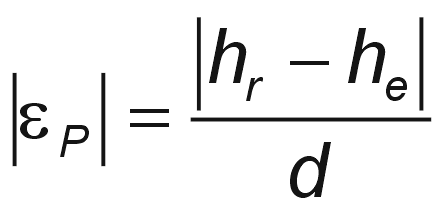where:

 d link distance (km) hr, he antenna heights above sea level (m)

## 1.6. Fresnel zones calculation

The position of obstacles between points of the bridge can significantly influence the quality of the microwave link. The radio signal doesn’t only radiate along the line of sight, but also in the area around it, i.e. in the so-called 1st Fresnel zone. Within this zone 90 % of the energy is transmitted between the transmitter and receiver antenna. This space has the shape of an ellipsoid. If it is disturbed the link has poorer transmission properties and a higher quality antenna is required. For this reason the position of the antenna can be just as important as its height above ground. 60 % of the 1st Fresnel zone is considered as the most important.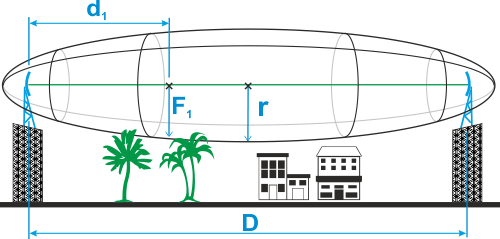Fig. 1.6: Fresnel zone

The general equation for calculating the first Fresnel zone radius at any point P in between the endpoints of the link is the following: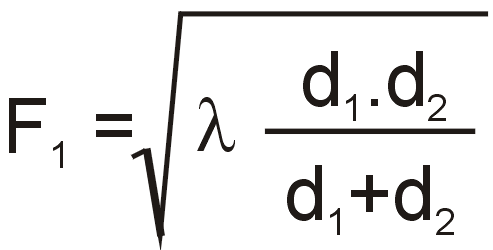Where:

 F1 first Fresnel Zone radius in metres d1 distance of P from one end in metres d2 The distance of P from the other end in metres λ wavelength of the transmitted signal in metres

The cross sectional radius of each Fresnel zone is the highest in the center of link, shrinking to a point at the antenna on each end. For practical applications, it is often useful to know the maximum radius of the first Fresnel zone. From the above formula, calculation of the first Fresnel zone can be simplified to: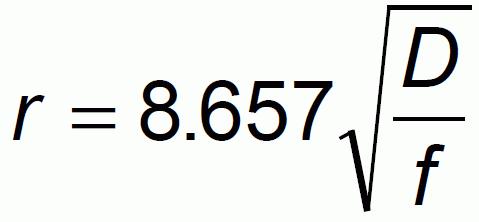where:

 r max radius of first Fresnel zone (m)reducing the radius to 60% get values ​​listed in the following table that define the space particularly sensitive to the presence of obstacles D total link distance (km) f frequency (GHz)

Tab. 1.3: 60 % of the 1st Fresnel zone

 Length of link D Radius of zone r for frequency 11 GHz 17 GHz 24 GHz 0,5 km 1.10 m 0.89 m 0.75 m 1 km 1.56 m 1.25 m 1.06 m 2 km 2.21 m 1.77 m 1.50 m 4 km 3.13 m 2.50 m 2.12 m 6 km 3.84 m 3.07 m 2.60 m 8 km 4.43 m 3.54 m 3.00 m 10 km 4.95 m 3.96 m 3.35 m 15 km 6.06 m 4.85 m 4.10 m 20 km 7.00 m 5.60 m 4.74 m 50 km 11.07 m